# R S Aggarwal Solutions for Class 10 Maths Chapter 5 Arithmetic Progression Exercise 5A

R S Aggarwal Solutions for Class 10 Maths exercise 5a are given here. To score good marks in exams, students are advised to solve all the questions provided in this exercise. Download pdf of Class 10 Maths Chapter 5 R S Aggarwal Solutions and practice offline. This exercise concept helps students to understand the arithmetic series, how to find the general term of an AP and the nth term from the end of an AP.

## Download PDF of R S Aggarwal Solutions for Class 10 Maths Chapter 5 Arithmetic Progression Exercise 5A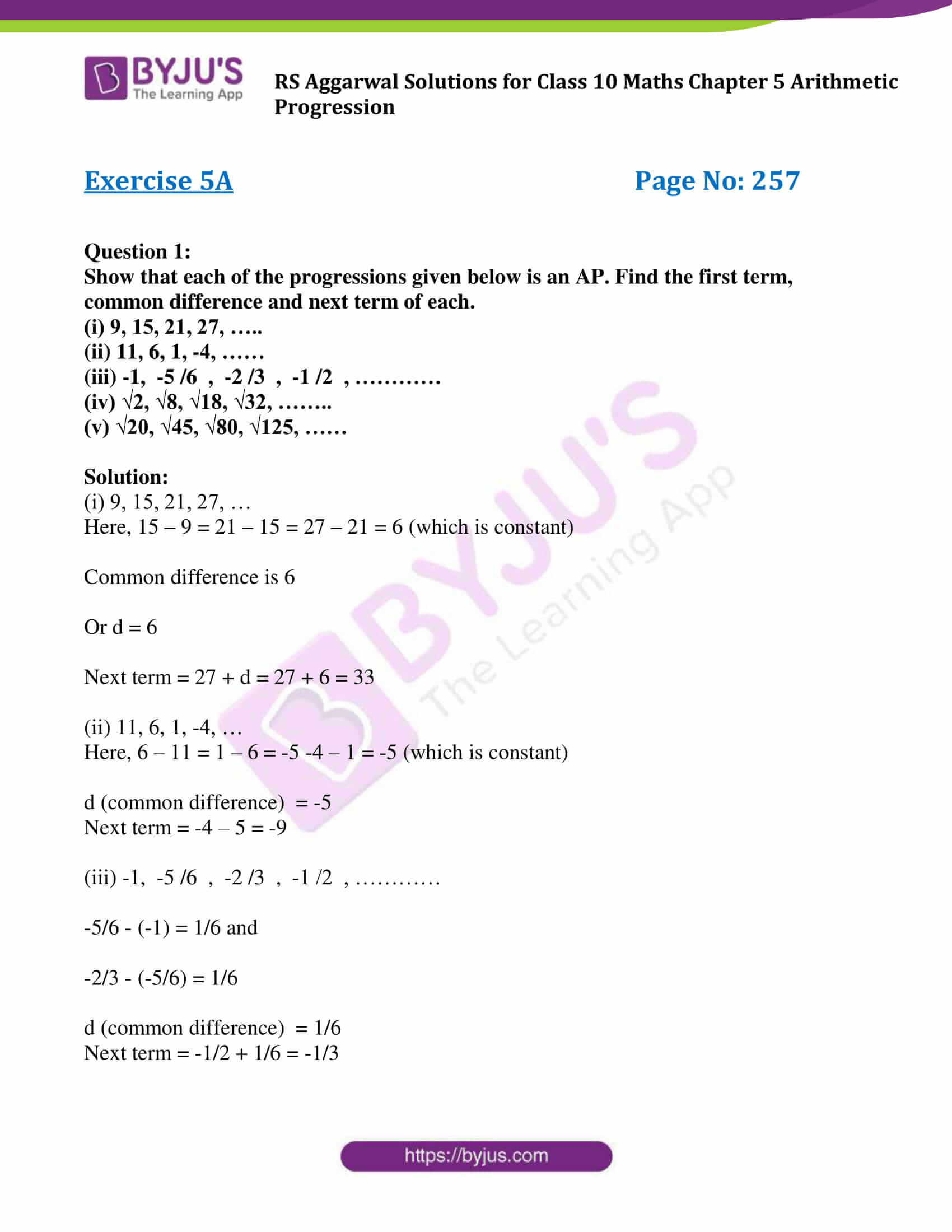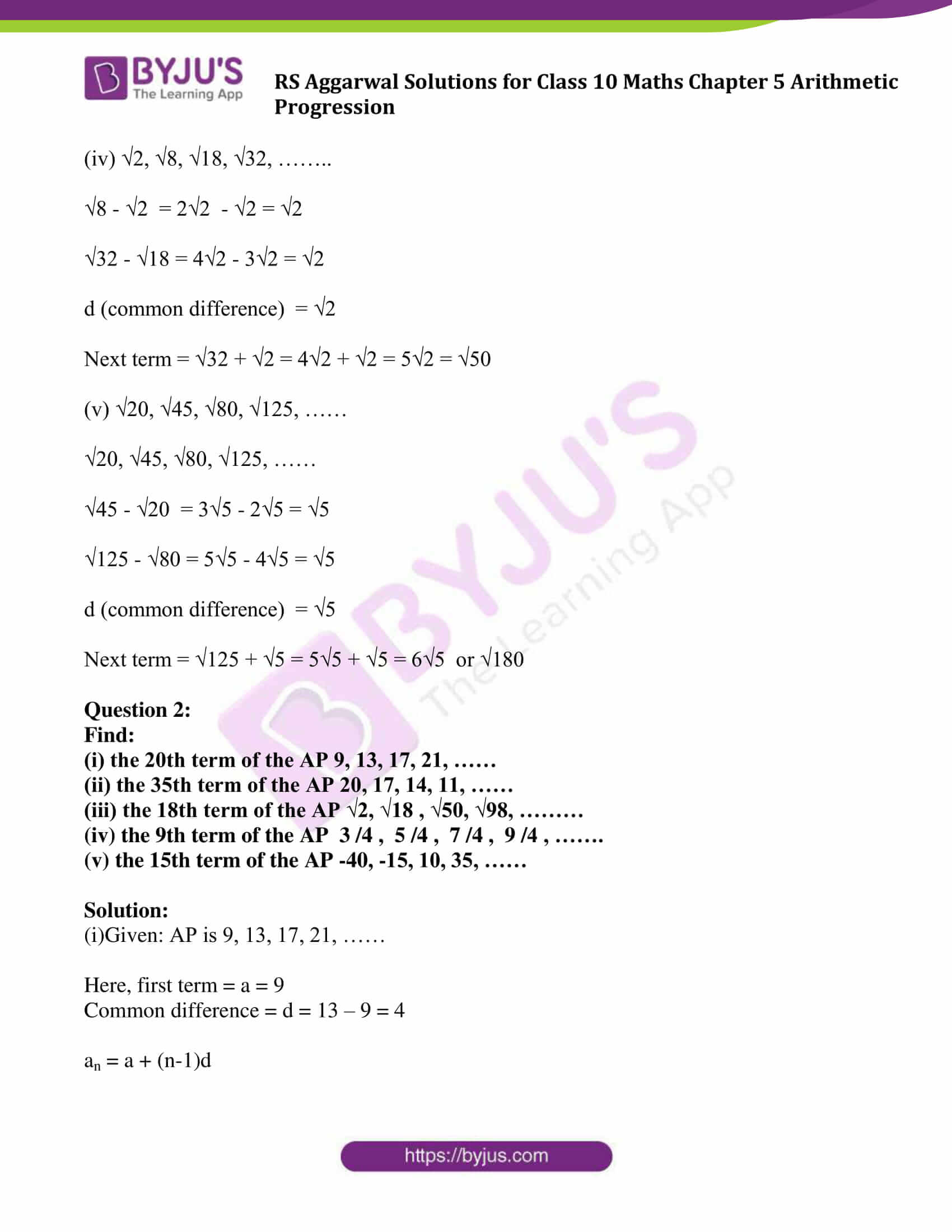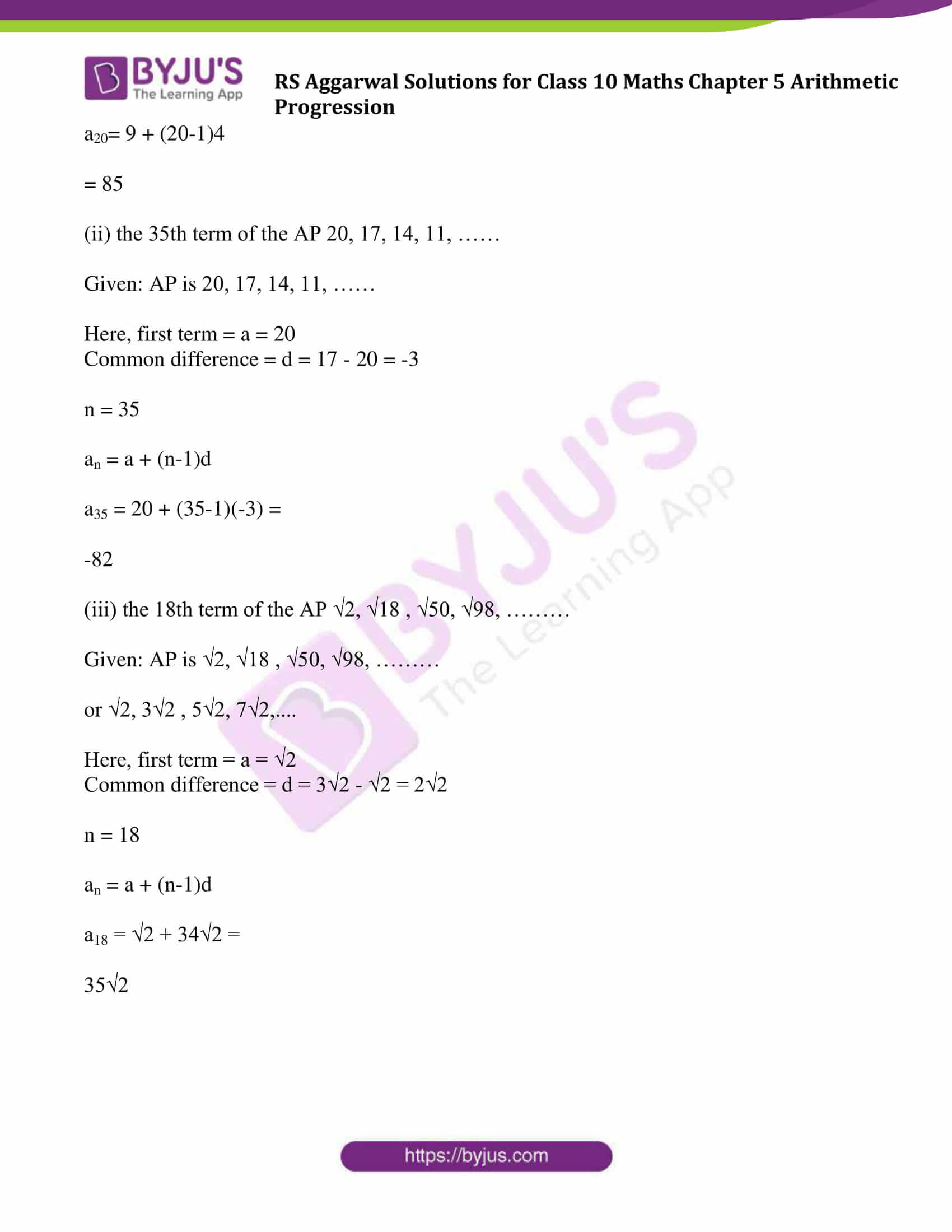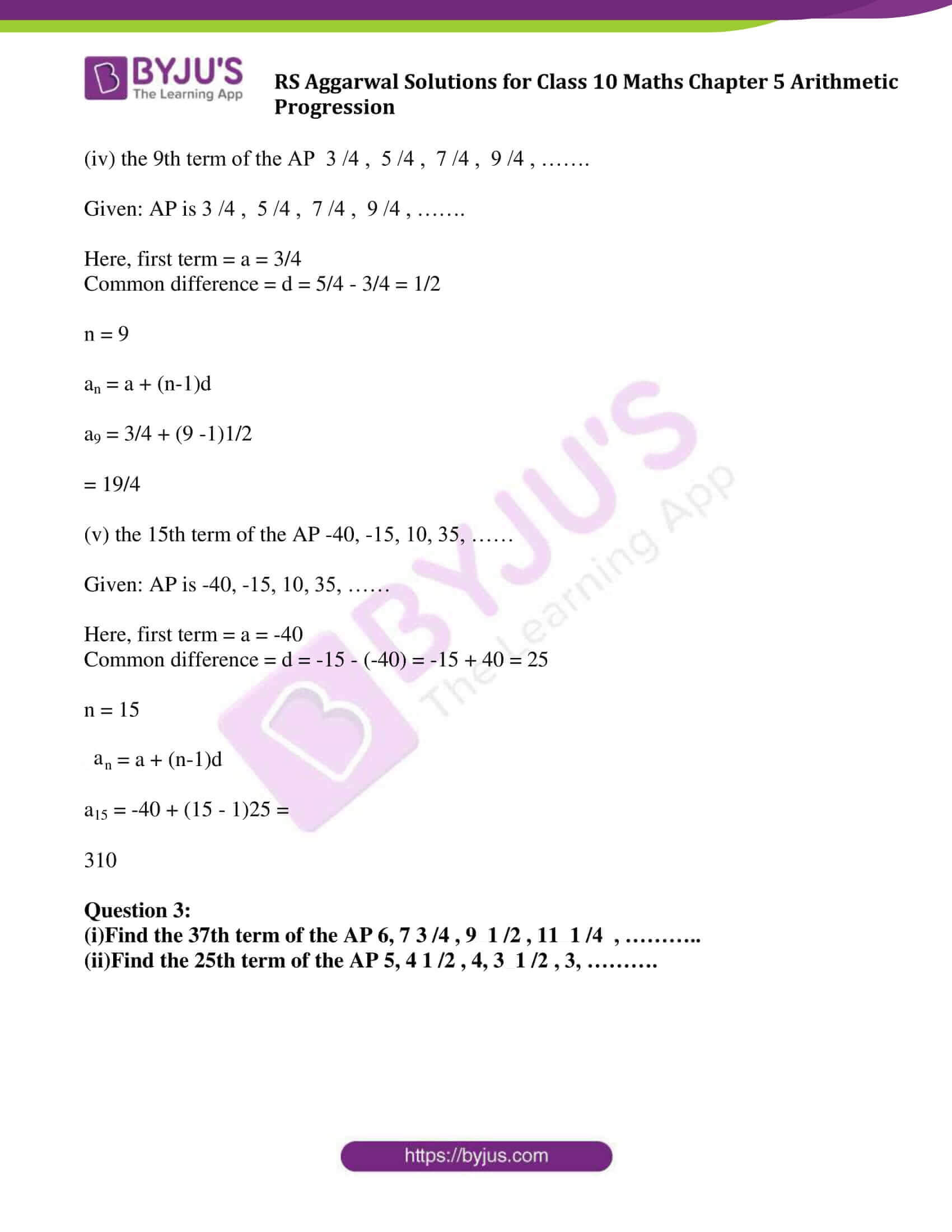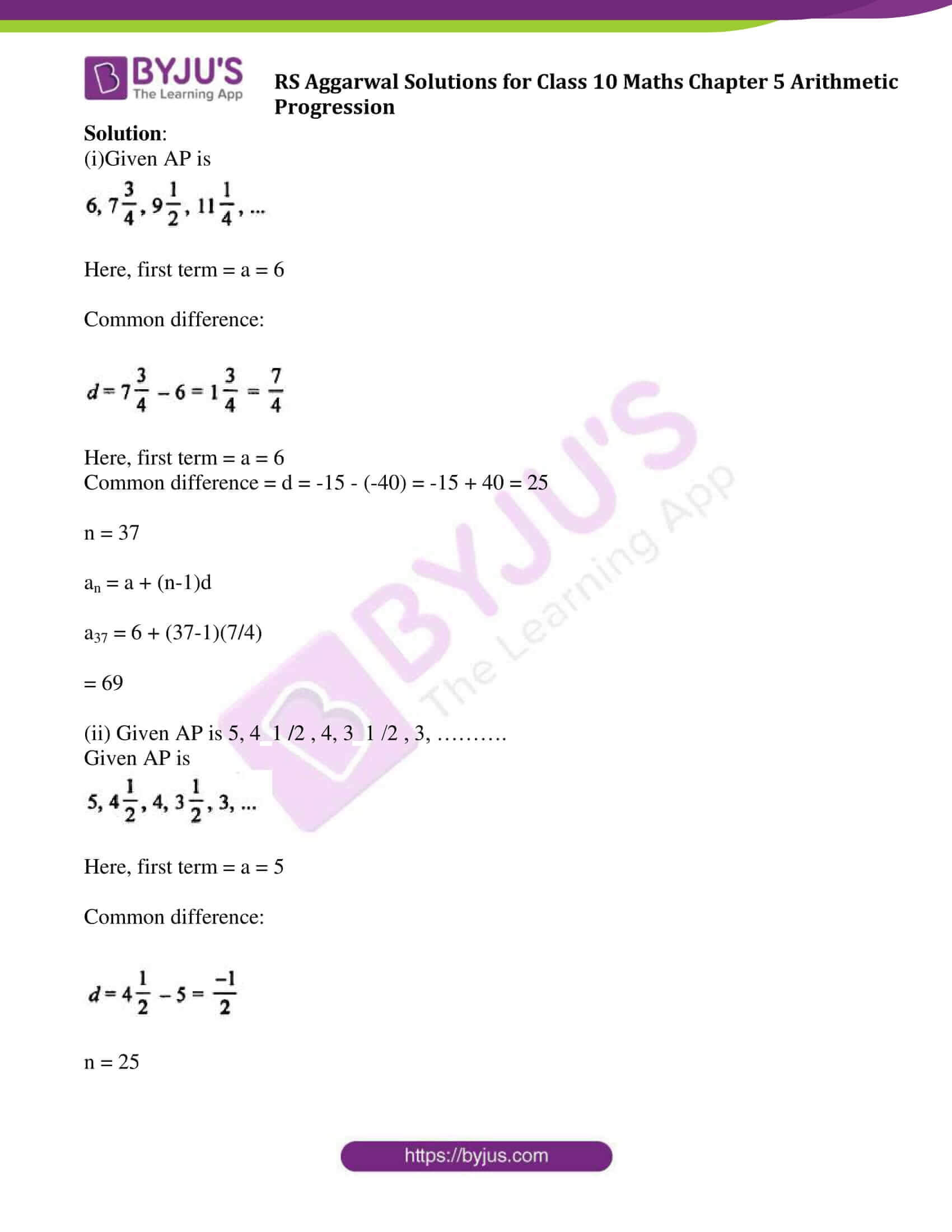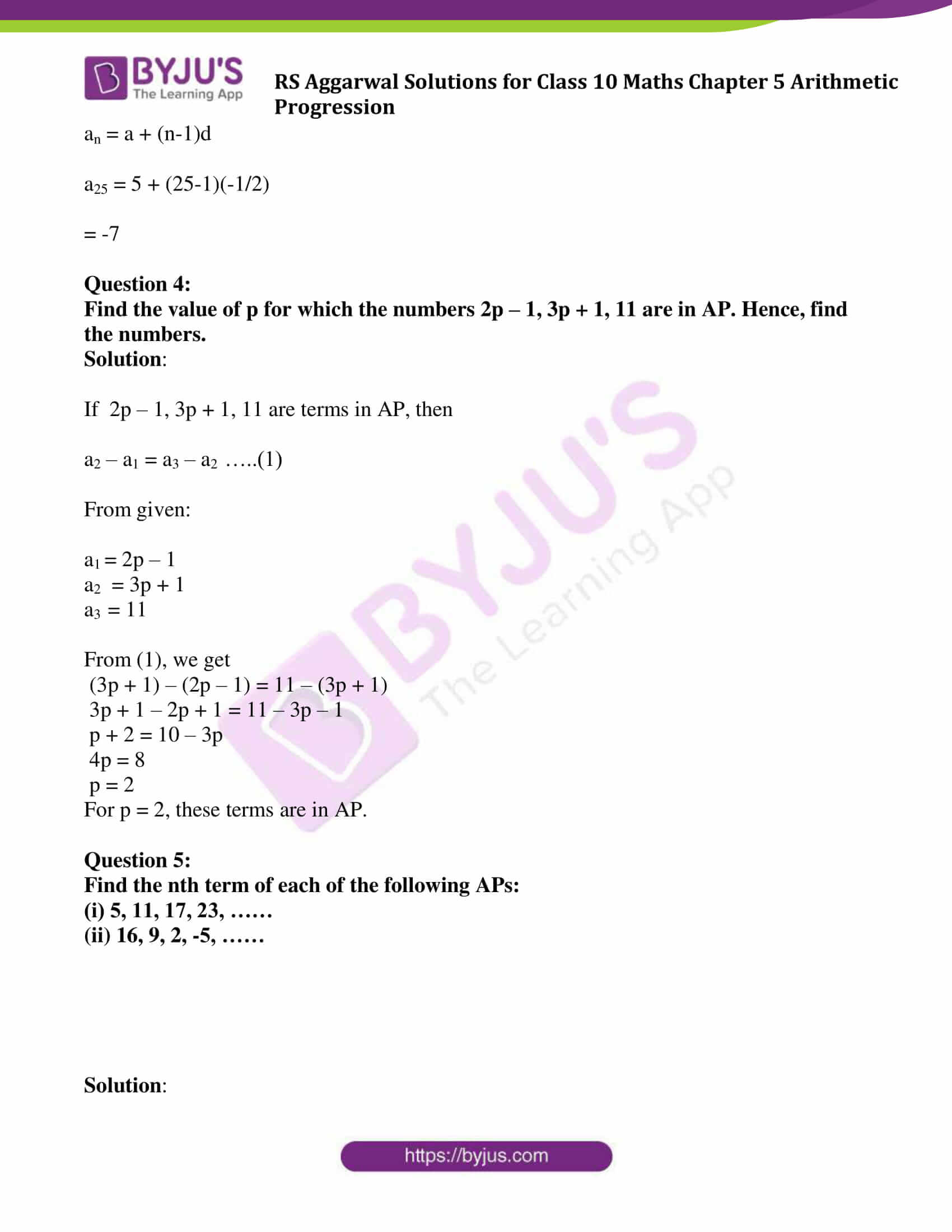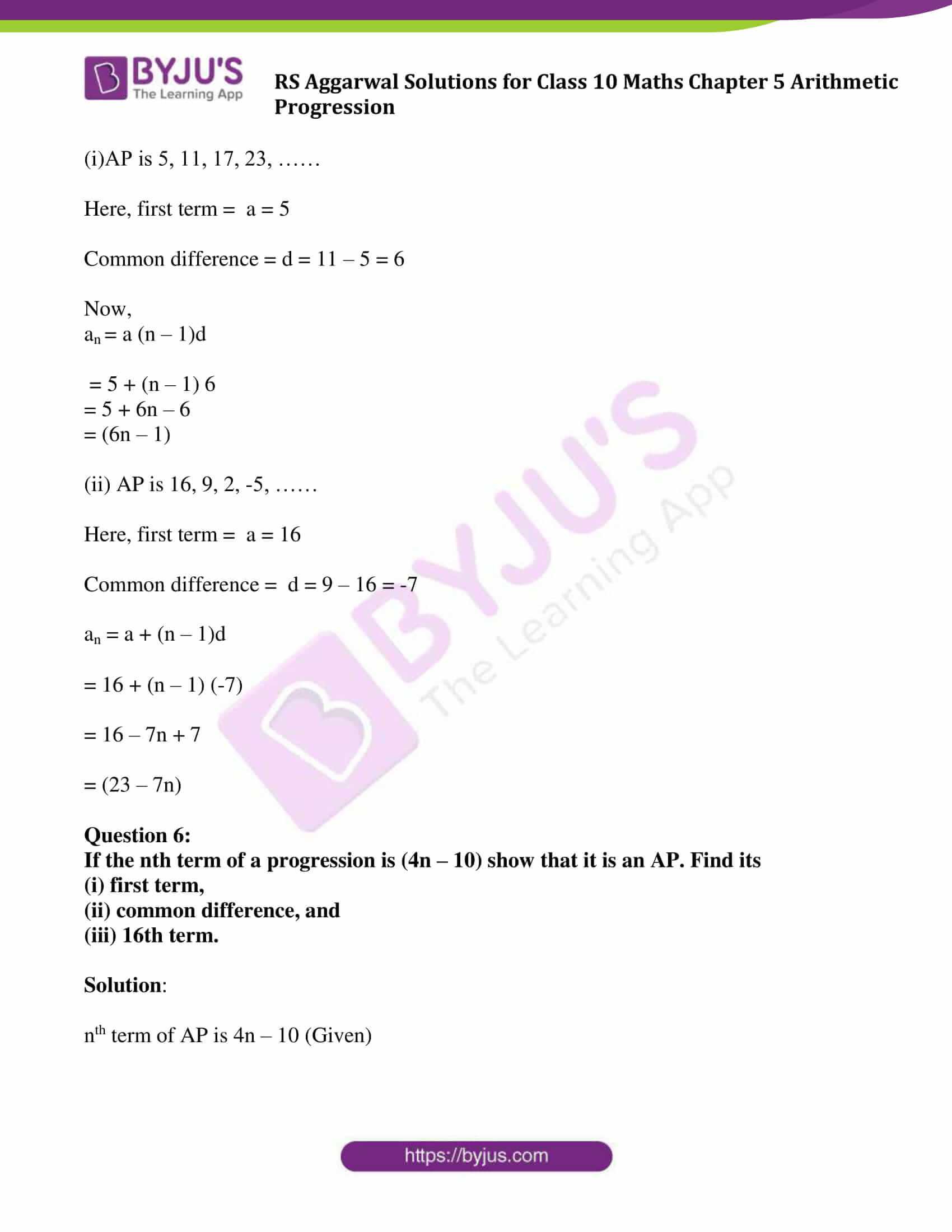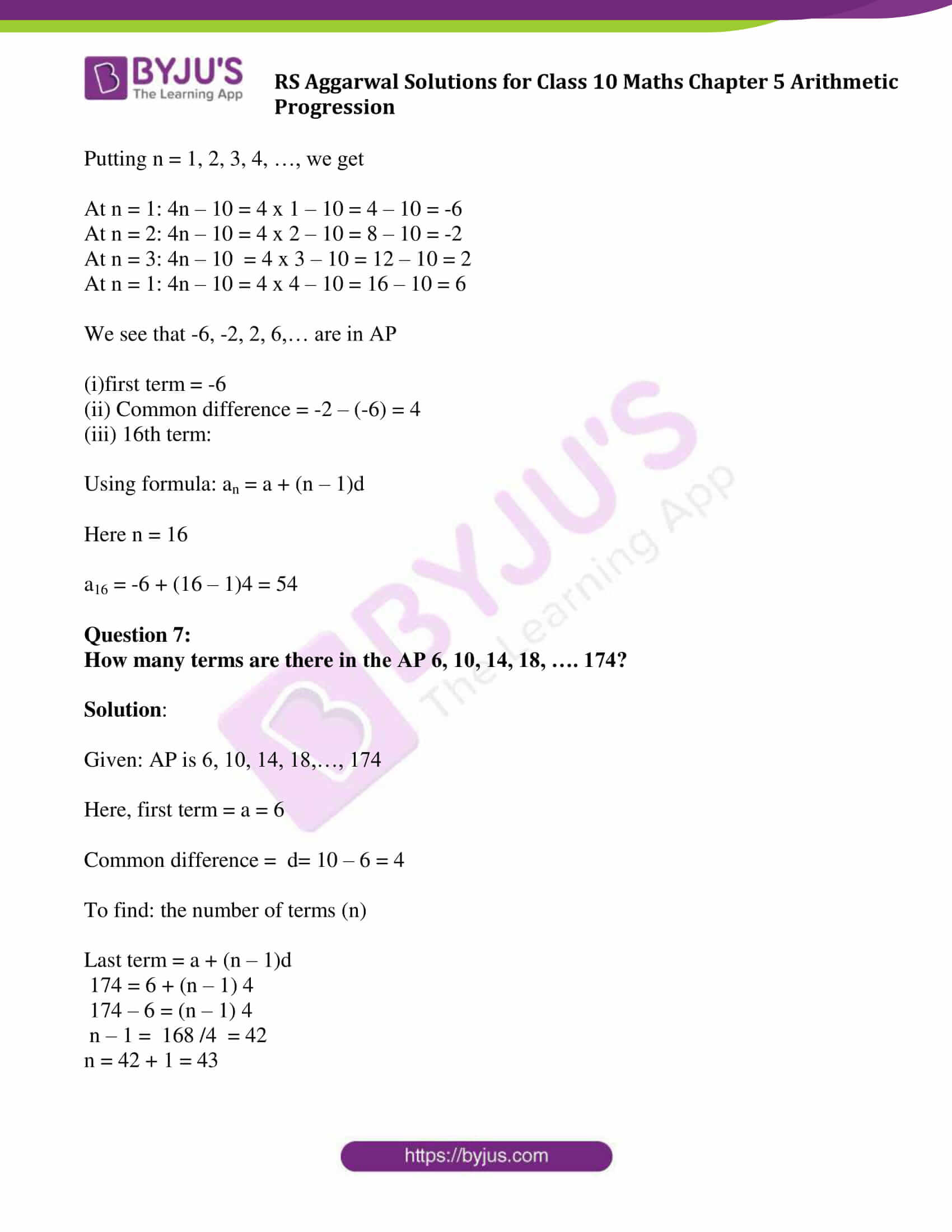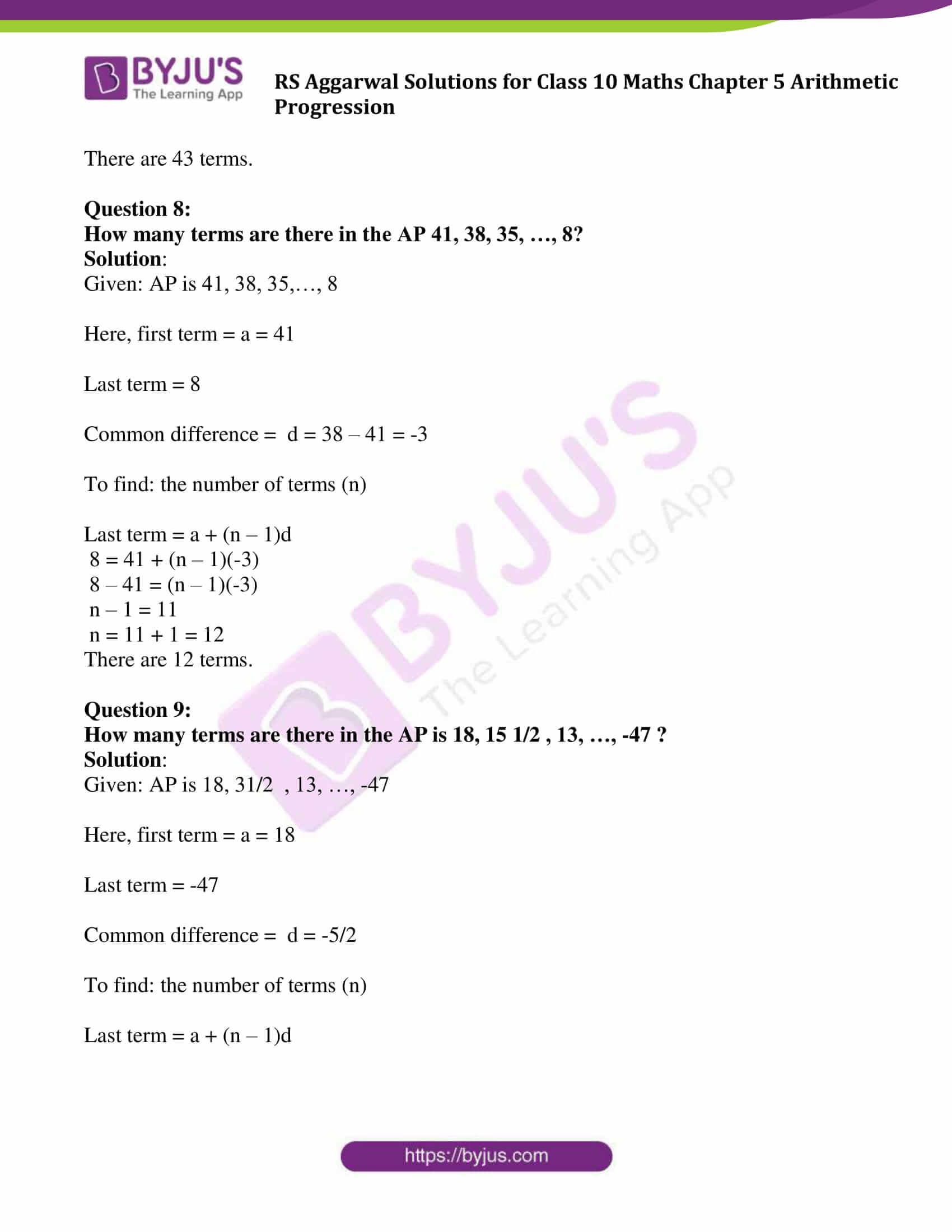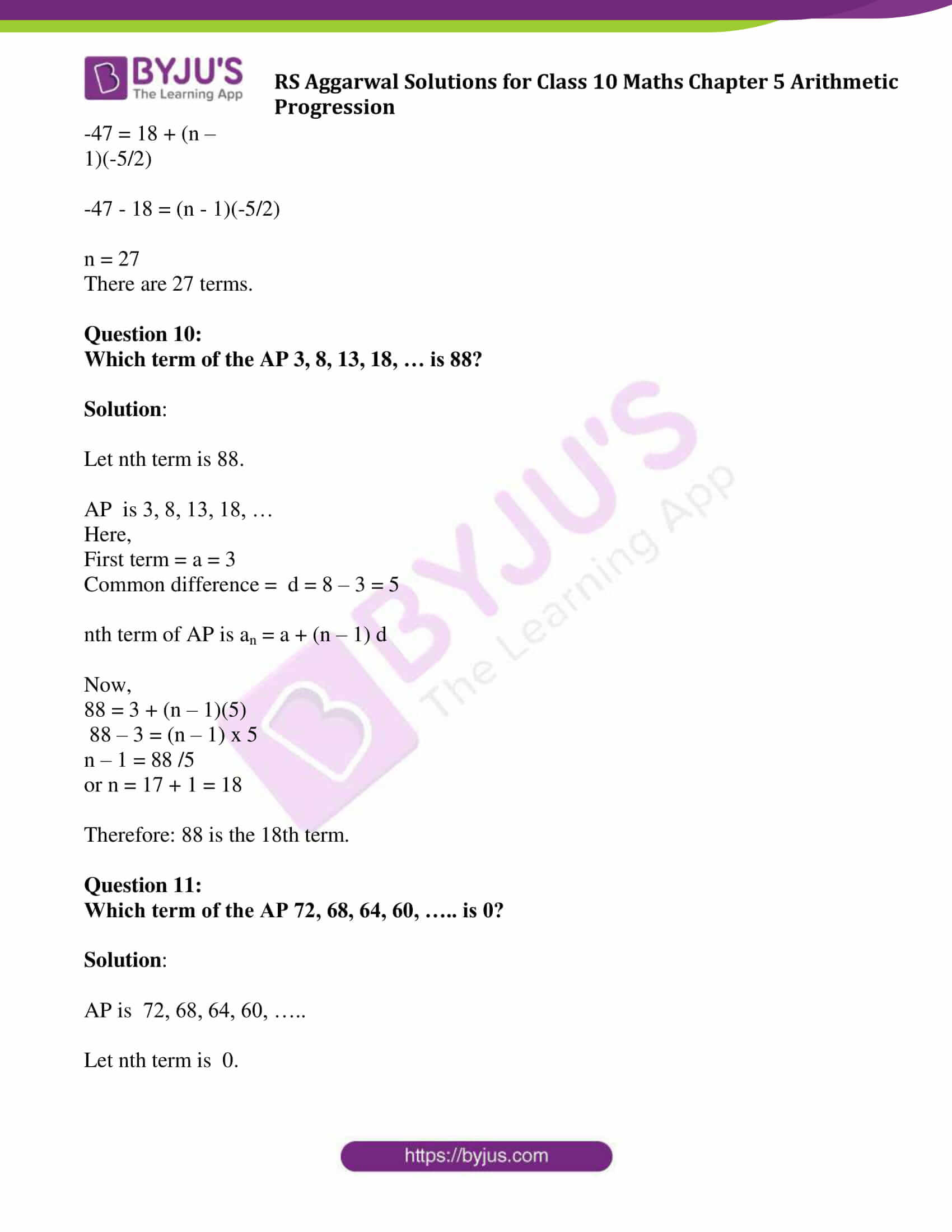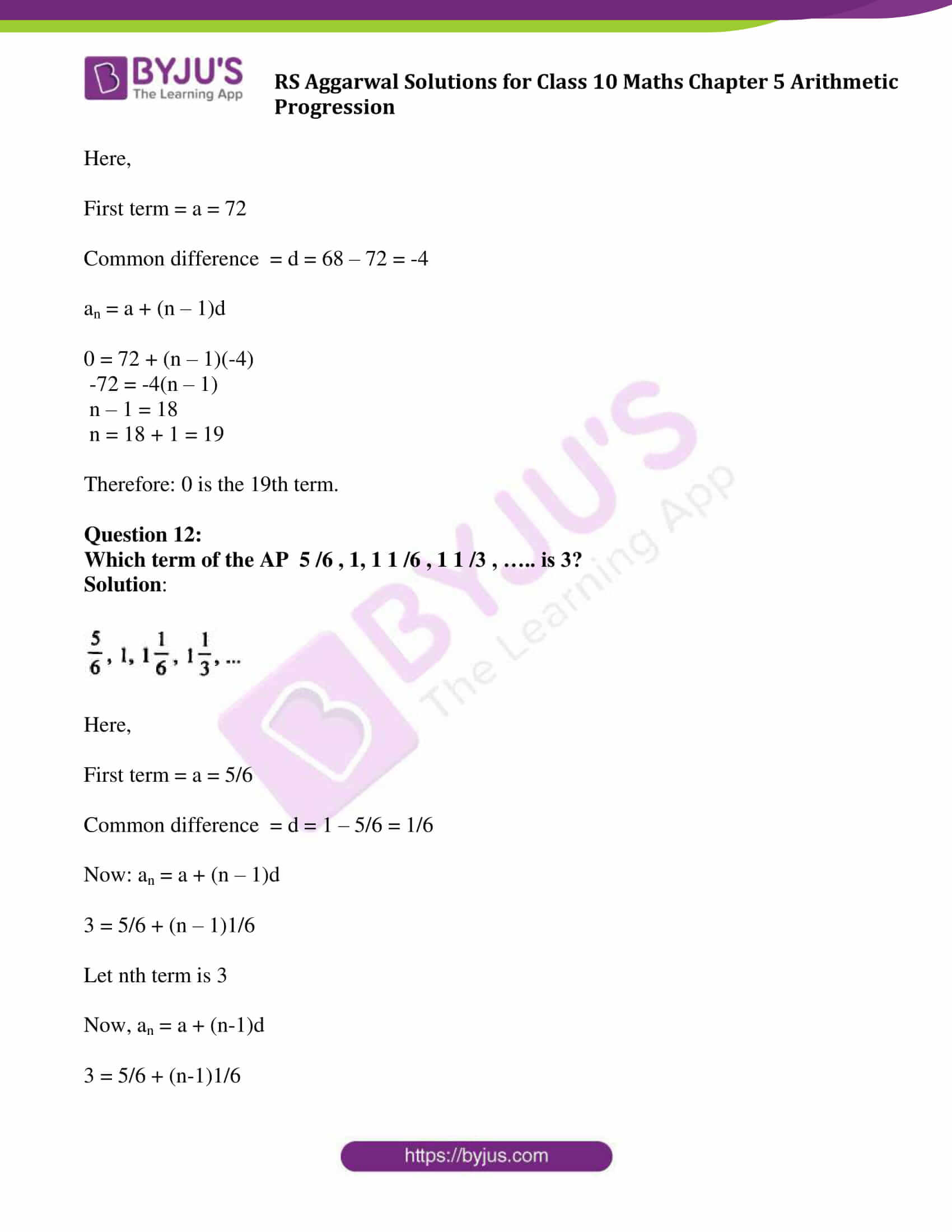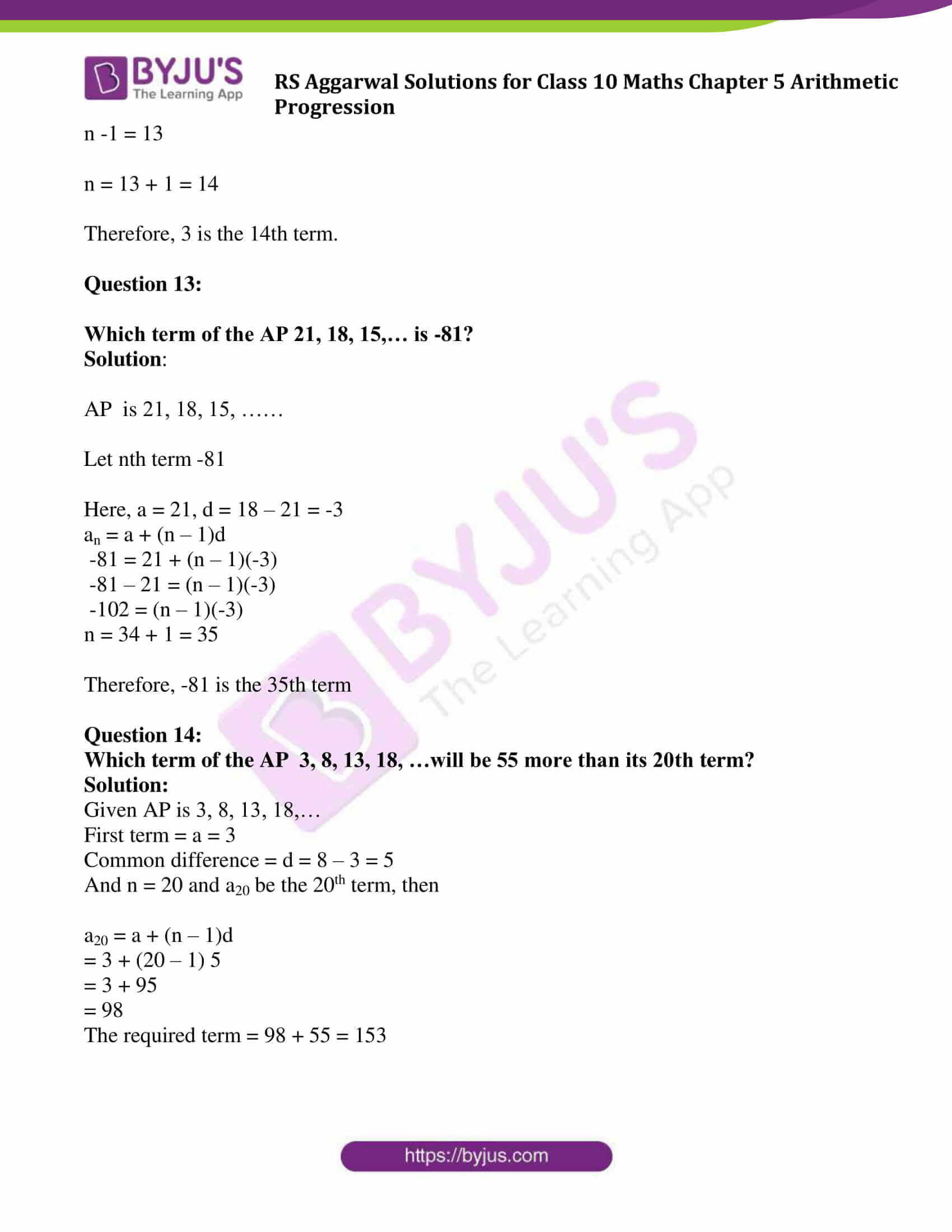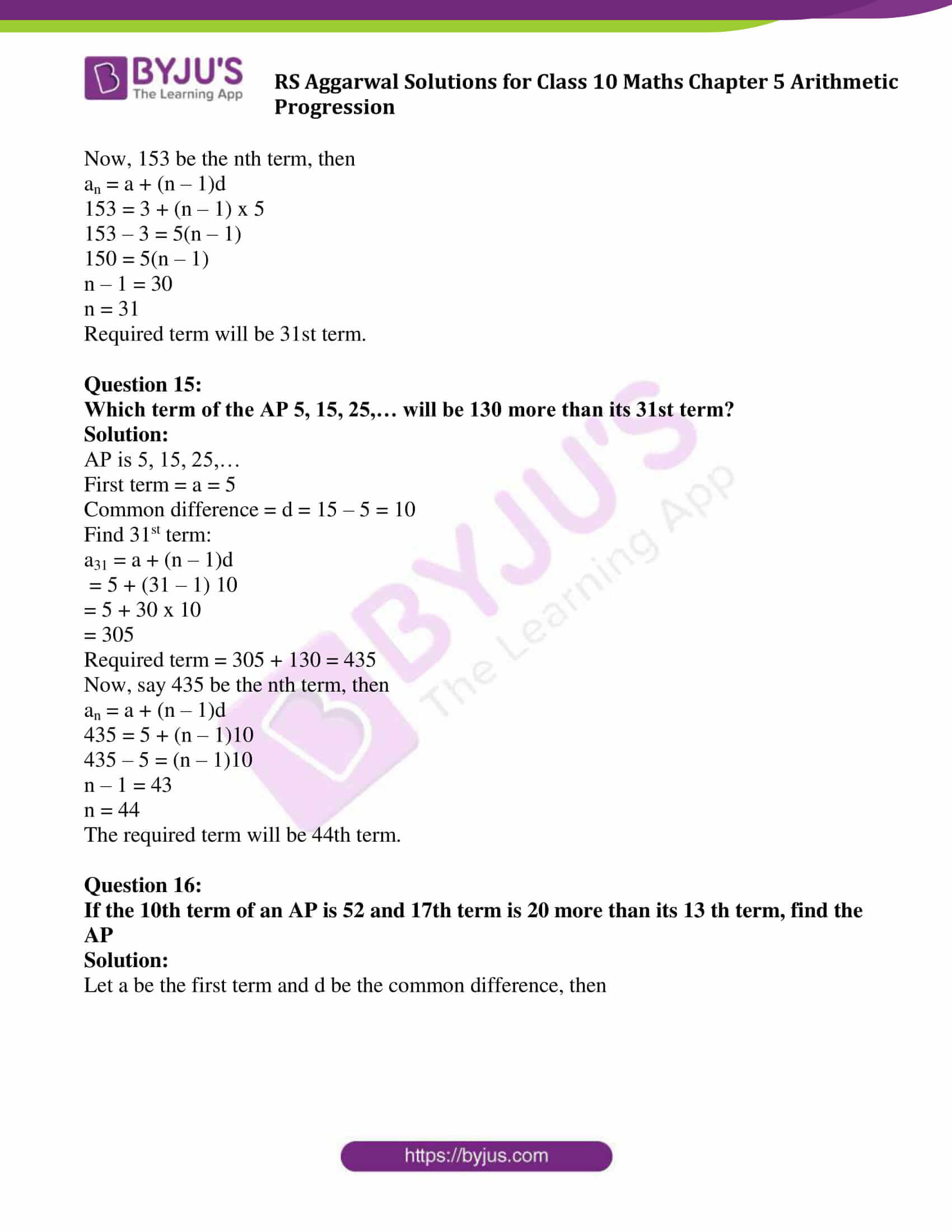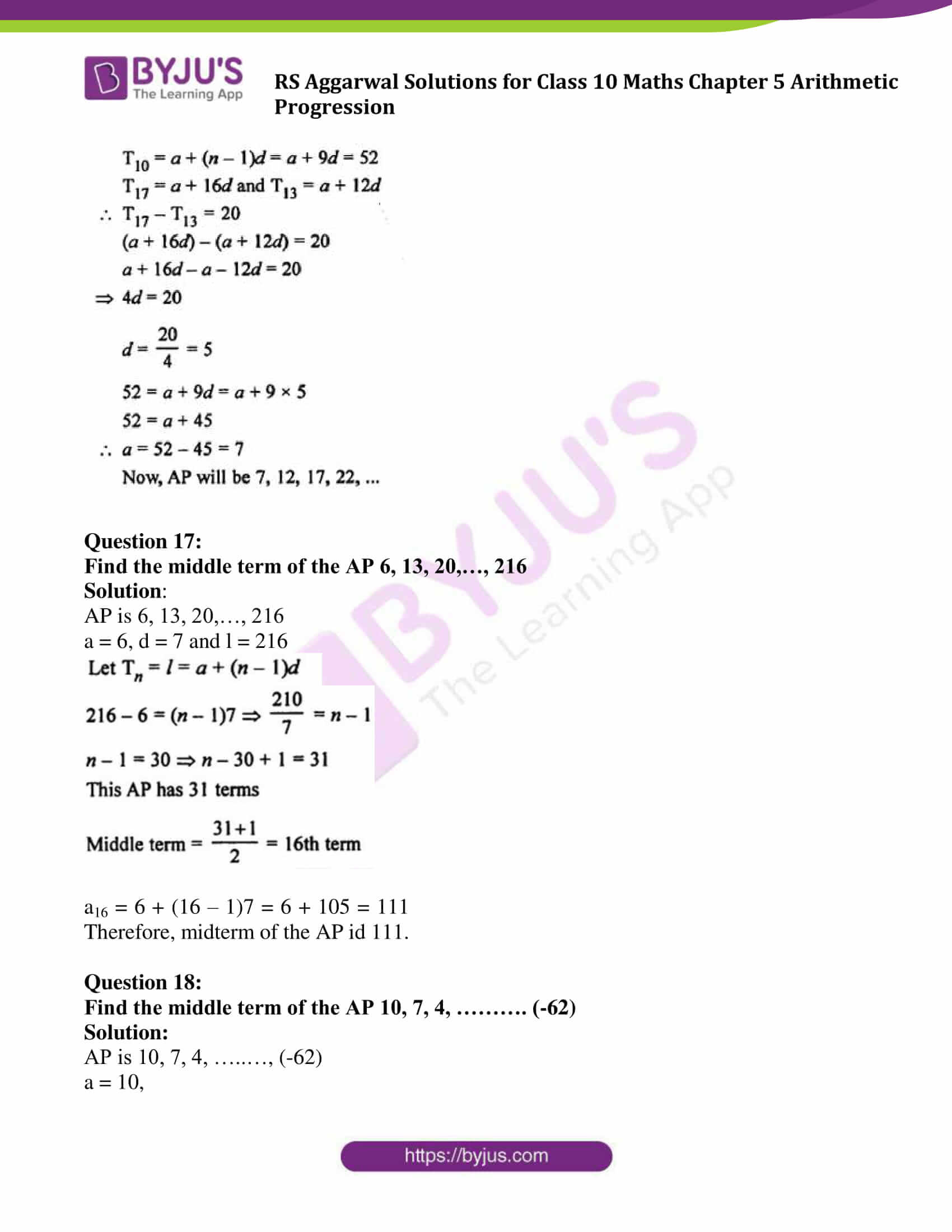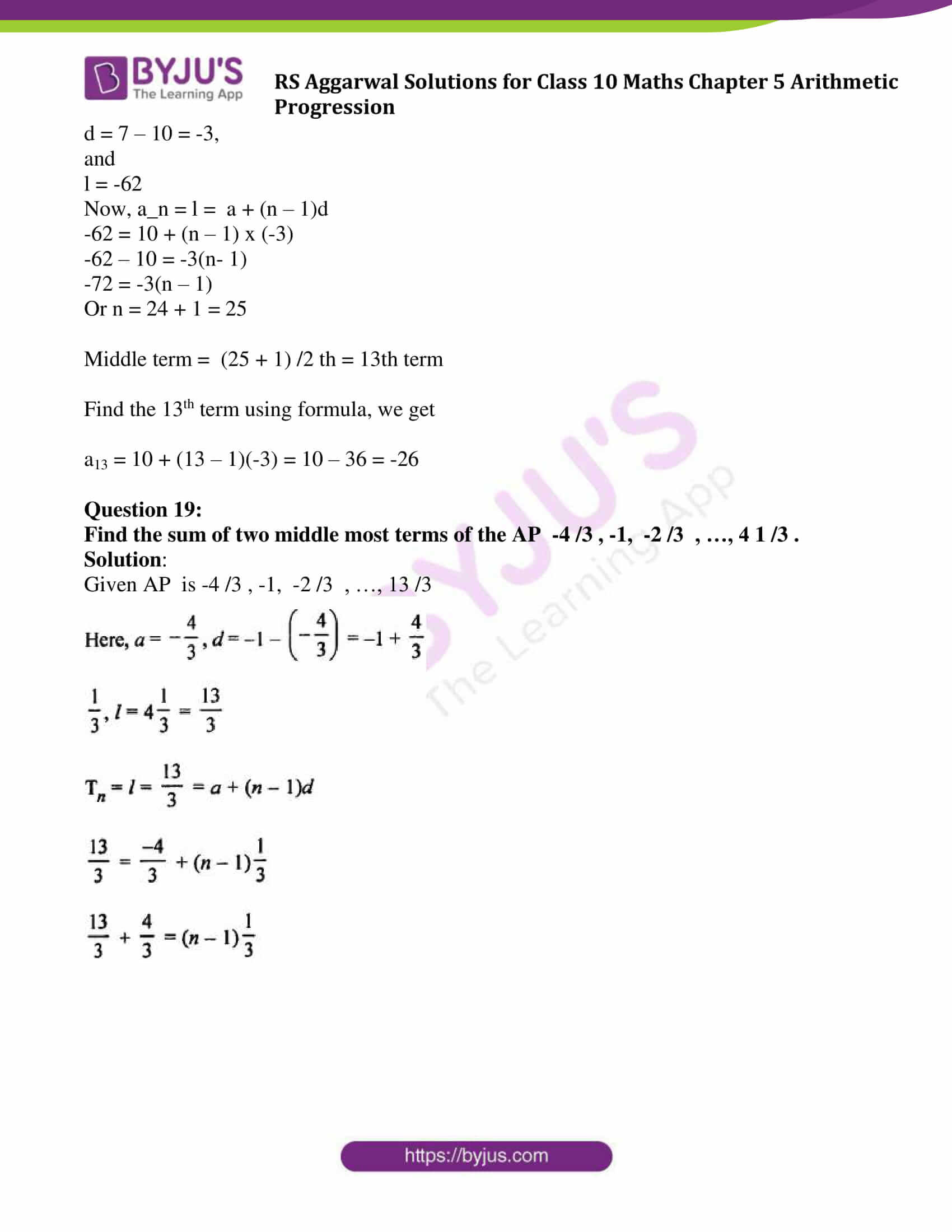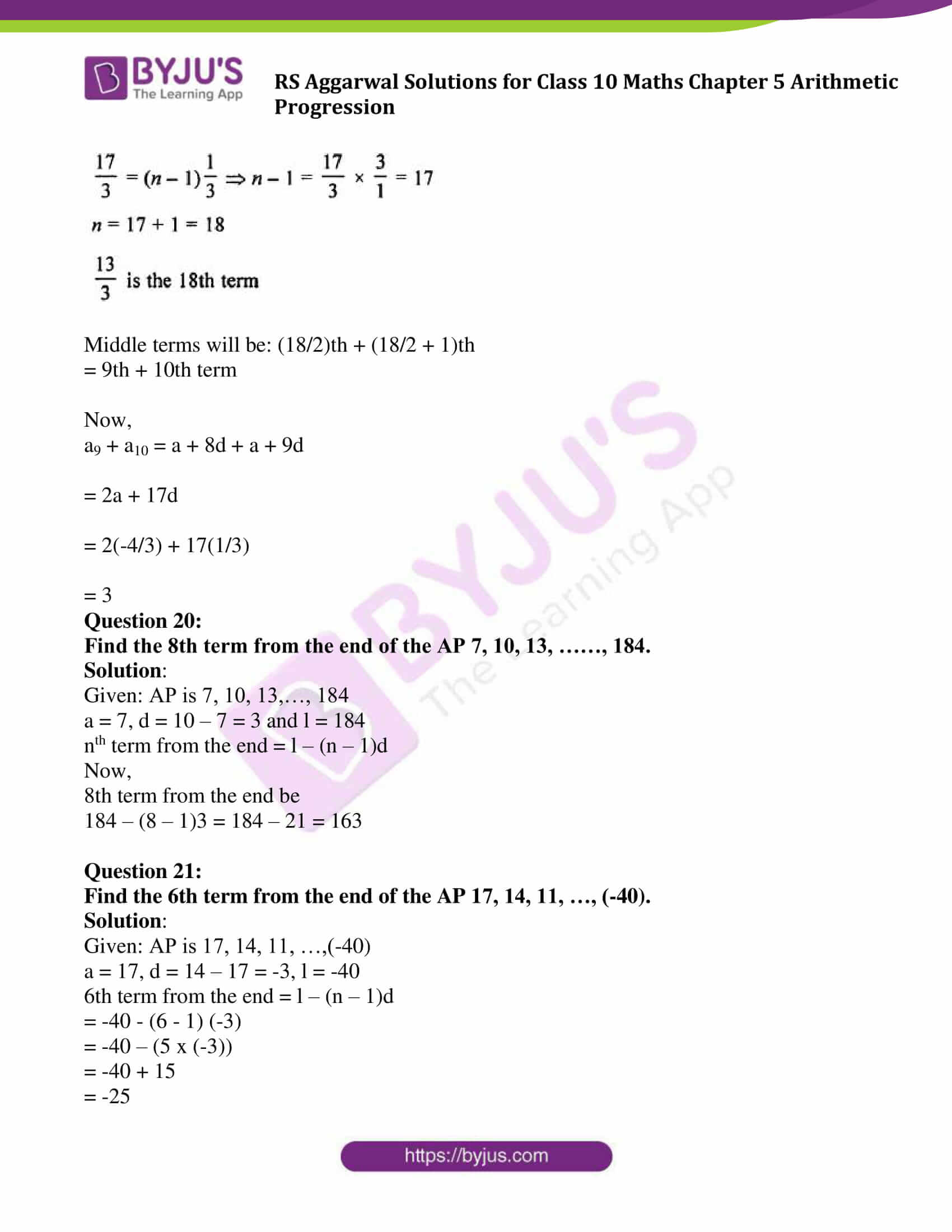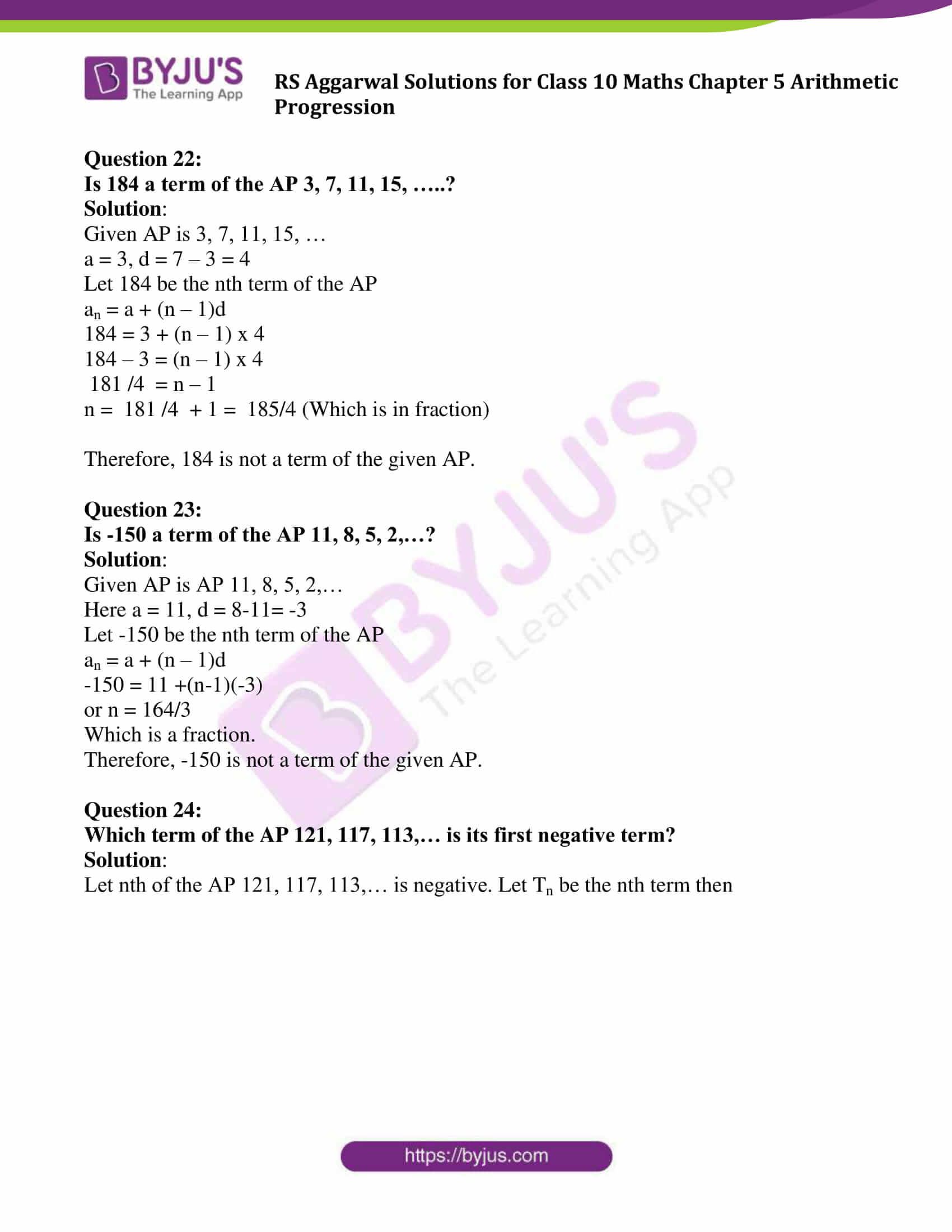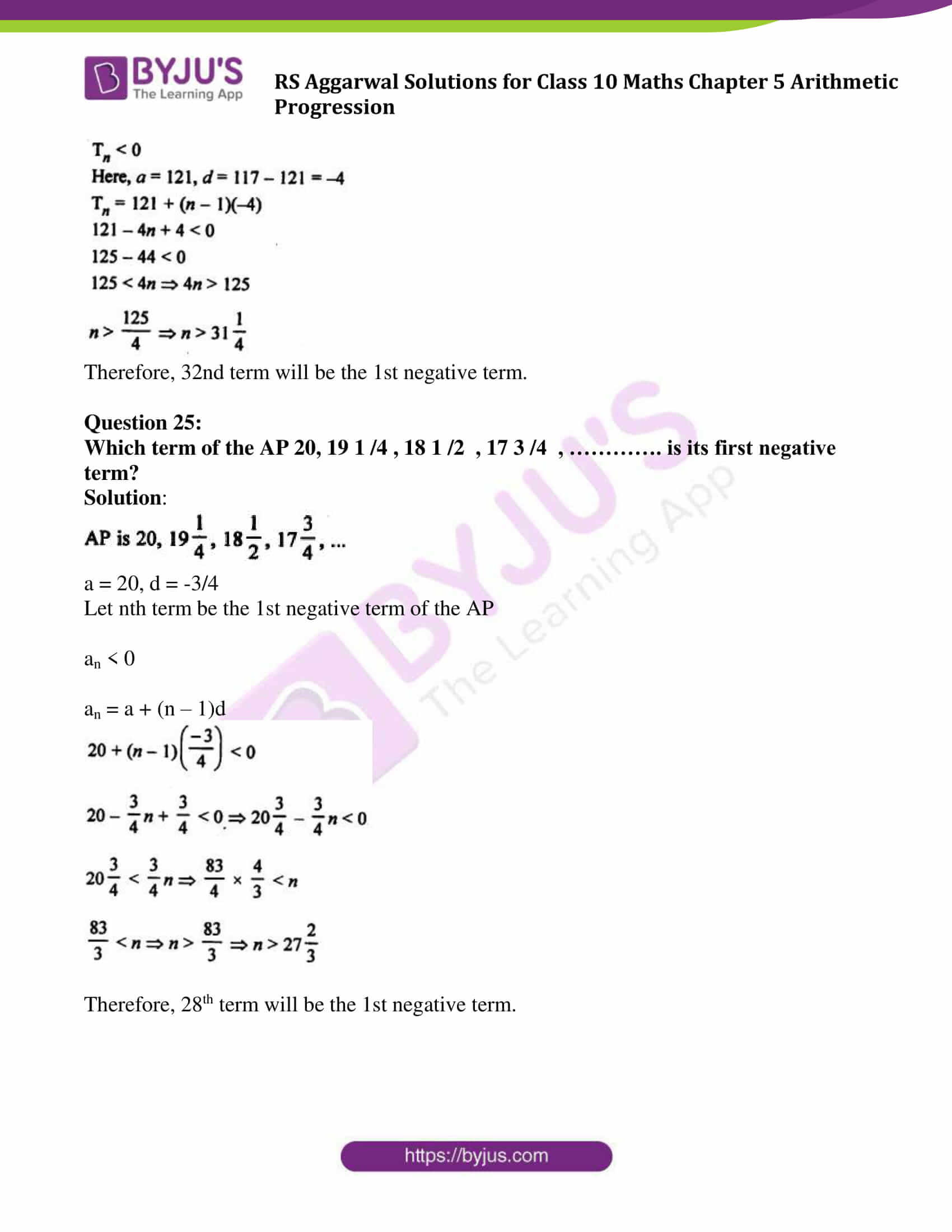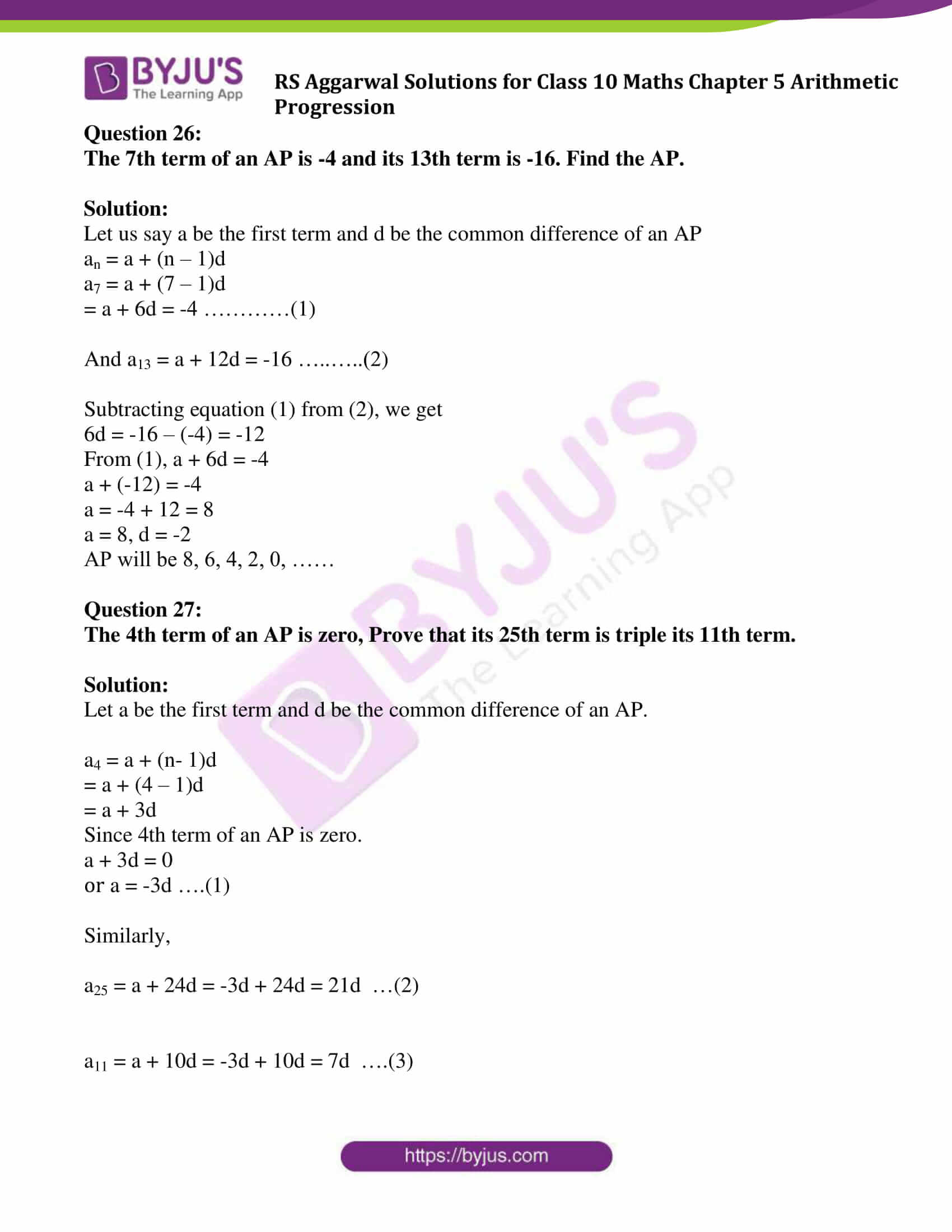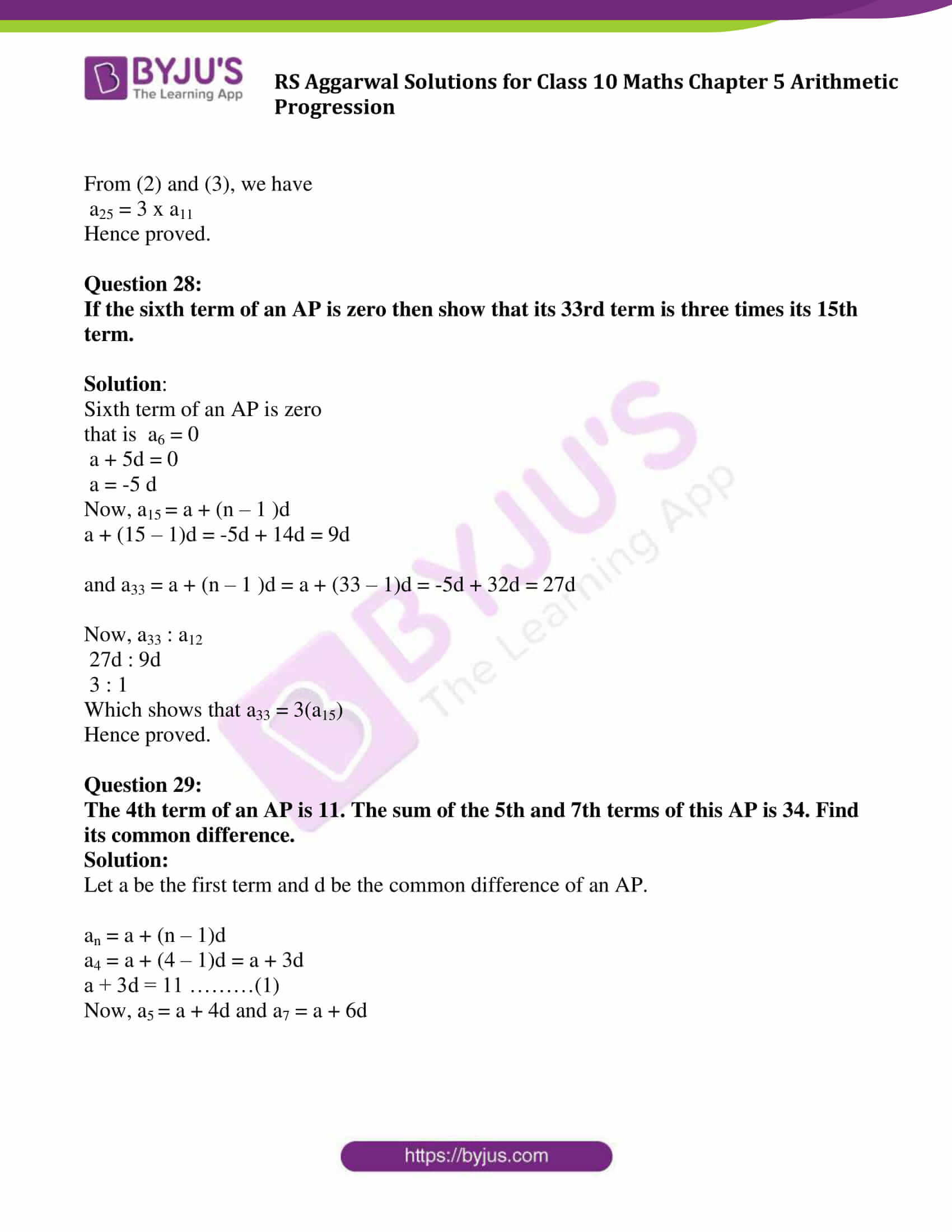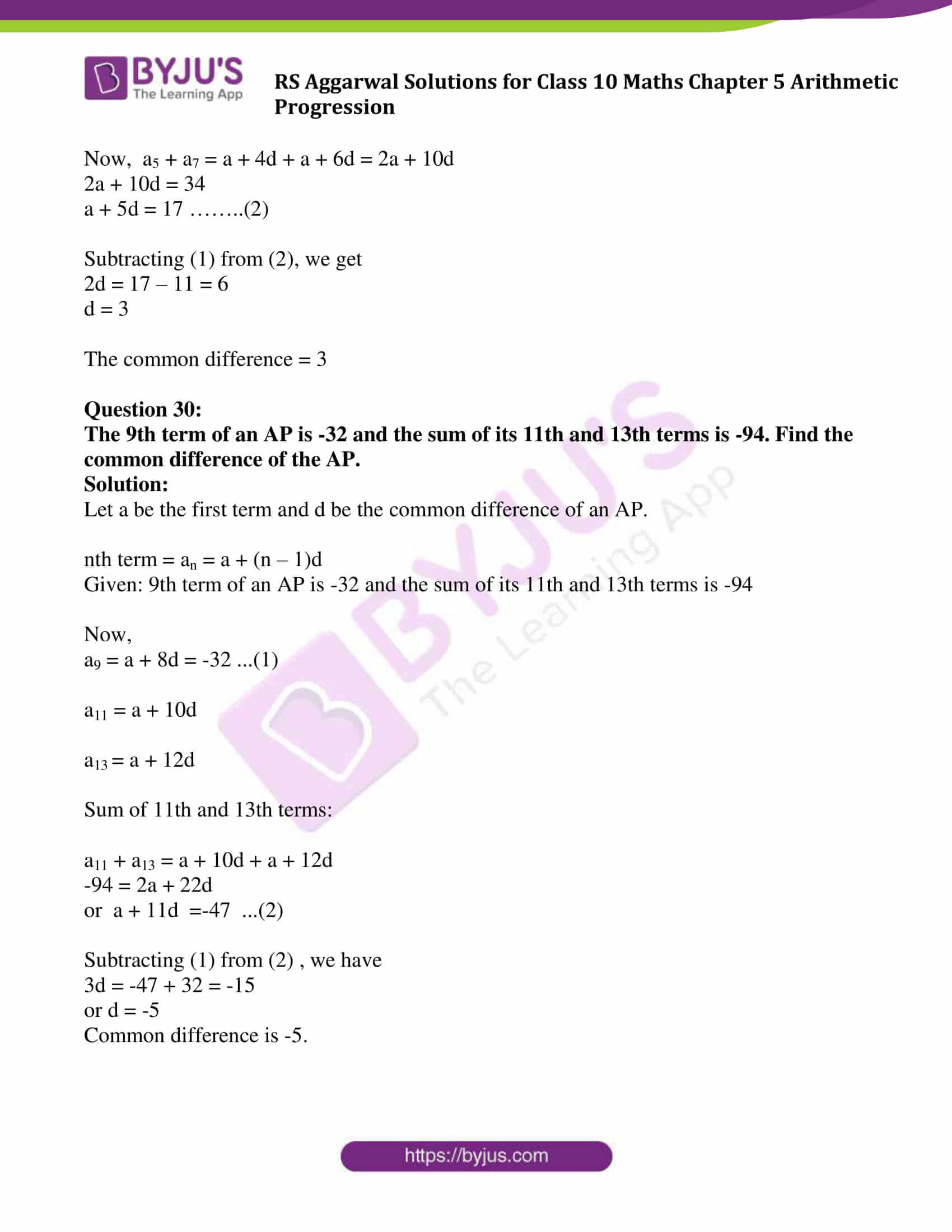### Access other exercise solutions of Class 10 Maths Chapter 5 Arithmetic Progression

Exercise 5B Solutions: 16 Questions (Short Answers)

Exercise 5C Solutions: 15 Questions (Short Answers)

Exercise 5D Solutions: 20 Questions (Long Answers)

## Exercise 5A Page No: 257

Question 1:

Show that each of the progressions given below is an AP. Find the first term, common difference and next term of each.

(i) 9, 15, 21, 27, …..

(ii) 11, 6, 1, -4, ……

(iii) -1, -5 /6 , -2 /3 , -1 /2 , …………

(iv) √2, √8, √18, √32, ……..

(v) √20, √45, √80, √125, ……

Solution:

(i) 9, 15, 21, 27, …

Here, 15 – 9 = 21 – 15 = 27 – 21 = 6 (which is constant)

Common difference is 6

Or d = 6

Next term = 27 + d = 27 + 6 = 33

(ii) 11, 6, 1, -4, …

Here, 6 – 11 = 1 – 6 = -5 -4 – 1 = -5 (which is constant)

d (common difference) = -5

Next term = -4 – 5 = -9

(iii) -1, -5 /6 , -2 /3 , -1 /2 , …………

-5/6 – (-1) = 1/6 and

-2/3 – (-5/6) = 1/6

d (common difference) = 1/6

Next term = -1/2 + 1/6 = -1/3

(iv) √2, √8, √18, √32, ……..

√8 – √2 = 2√2 – √2 = √2

√32 – √18 = 4√2 – 3√2 = √2

d (common difference) = √2

Next term = √32 + √2 = 4√2 + √2 = 5√2 = √50

(v) √20, √45, √80, √125, ……

√20, √45, √80, √125, ……

√45 – √20 = 3√5 – 2√5 = √5

√125 – √80 = 5√5 – 4√5 = √5

d (common difference) = √5

Next term = √125 + √5 = 5√5 + √5 = 6√5 or √180

Question 2:

Find:

(i) the 20th term of the AP 9, 13, 17, 21, ……

(ii) the 35th term of the AP 20, 17, 14, 11, ……

(iii) the 18th term of the AP √2, √18 , √50, √98, ………

(iv) the 9th term of the AP 3 /4 , 5 /4 , 7 /4 , 9 /4 , …….

(v) the 15th term of the AP -40, -15, 10, 35, ……

Solution:

(i)Given: AP is 9, 13, 17, 21, ……

Here, first term = a = 9

Common difference = d = 13 – 9 = 4

an = a + (n-1)d

a20= 9 + (20-1)4

= 85

(ii) the 35th term of the AP 20, 17, 14, 11, ……

Given: AP is 20, 17, 14, 11, ……

Here, first term = a = 20

Common difference = d = 17 – 20 = -3

n = 35

an = a + (n-1)d

a35 = 20 + (35-1)(-3)

= -82

(iii) the 18th term of the AP √2, √18 , √50, √98, ………

Given: AP is √2, √18 , √50, √98, ………

or √2, 3√2 , 5√2, 7√2,….

Here, first term = a = √2

Common difference = d = 3√2 – √2 = 2√2

n = 18

an = a + (n-1)d

a18 = √2 + 34√2

= 35√2

(iv) the 9th term of the AP 3 /4 , 5 /4 , 7 /4 , 9 /4 , …….

Given: AP is 3 /4 , 5 /4 , 7 /4 , 9 /4 , …….

Here, first term = a = 3/4

Common difference = d = 5/4 – 3/4 = 1/2

n = 9

an = a + (n-1)d

a9 = 3/4 + (9 -1)1/2

= 19/4

(v) the 15th term of the AP -40, -15, 10, 35, ……

Given: AP is -40, -15, 10, 35, ……

Here, first term = a = -40

Common difference = d = -15 – (-40) = -15 + 40 = 25

n = 15

an = a + (n-1)d

a15 = -40 + (15 – 1)25

= 310

Question 3:

(i) Find the 37th term of the AP 6, $7\frac{3}{4}$ , $9\frac{1}{2}$ , $11\frac{1}{4}$, ………..

(ii) Find the 25th term of the AP 5, $4\frac{1}{2}$ , 4, $3\frac{1}{2}$ , 3, ……….

Solution: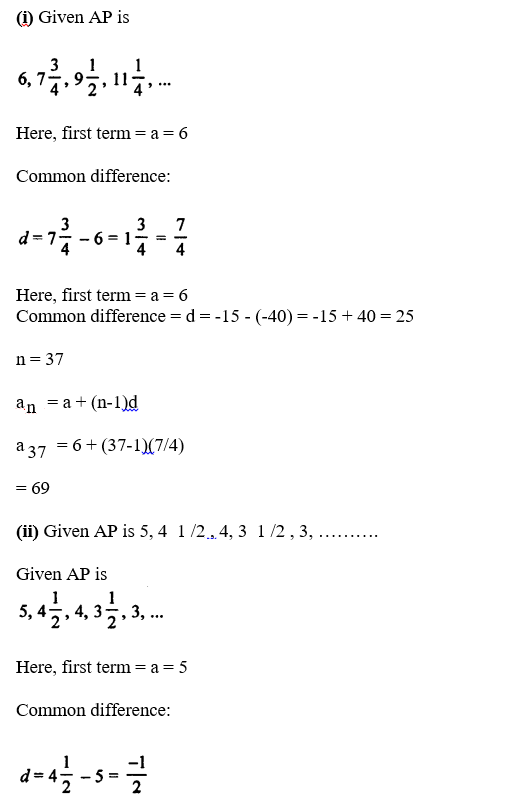n = 25

an = a + (n-1)d

a25 = 5 + (25-1)(-1/2)

= -7

Question 4:

Find the value of p for which the numbers 2p – 1, 3p + 1, 11 are in AP. Hence, find the numbers.

Solution:

If 2p – 1, 3p + 1, 11 are terms in AP, then

a2 – a1 = a3 – a2 …..(1)

From given:

a1 = 2p – 1

a2 = 3p + 1

a3 = 11

From (1), we get

(3p + 1) – (2p – 1) = 11 – (3p + 1)

3p + 1 – 2p + 1 = 11 – 3p – 1

p + 2 = 10 – 3p

4p = 8

p = 2

For p = 2, these terms are in AP.

Question 5:

Find the nth term of each of the following APs:

(i) 5, 11, 17, 23, ……

(ii) 16, 9, 2, -5, ……

Solution:

(i)AP is 5, 11, 17, 23, ……

Here, first term = a = 5

Common difference = d = 11 – 5 = 6

Now,

an = a (n – 1)d

= 5 + (n – 1) 6

= 5 + 6n – 6

= (6n – 1)

(ii) AP is 16, 9, 2, -5, ……

Here, first term = a = 16

Common difference = d = 9 – 16 = -7

an = a + (n – 1)d

= 16 + (n – 1) (-7)

= 16 – 7n + 7

= (23 – 7n)

Question 6:

If the nth term of a progression is (4n – 10) show that it is an AP. Find its

(i) first term,

(ii) common difference, and

(iii) 16th term.

Solution:

nth term of AP is 4n – 10 (Given)

Putting n = 1, 2, 3, 4, …, we get

At n = 1: 4n – 10 = 4 x 1 – 10 = 4 – 10 = -6

At n = 2: 4n – 10 = 4 x 2 – 10 = 8 – 10 = -2

At n = 3: 4n – 10 = 4 x 3 – 10 = 12 – 10 = 2

At n = 1: 4n – 10 = 4 x 4 – 10 = 16 – 10 = 6

We see that -6, -2, 2, 6,… are in AP

(i) first term = -6

(ii) Common difference = -2 – (-6) = 4

(iii) 16th term:

Using formula: an = a + (n – 1)d

Here n = 16

a16 = -6 + (16 – 1)4 = 54

Question 7:

How many terms are there in the AP 6, 10, 14, 18, …. 174?

Solution:

Given: AP is 6, 10, 14, 18,…, 174

Here, first term = a = 6

Common difference = d= 10 – 6 = 4

To find: the number of terms (n)

Last term = a + (n – 1)d

174 = 6 + (n – 1) 4

174 – 6 = (n – 1) 4

n – 1 = 168 /4 = 42

n = 42 + 1 = 43

There are 43 terms.

Question 8:

How many terms are there in the AP 41, 38, 35, …, 8?

Solution:

Given: AP is 41, 38, 35,…, 8

Here, first term = a = 41

Last term = 8

Common difference = d = 38 – 41 = -3

To find: the number of terms (n)

Last term = a + (n – 1)d

8 = 41 + (n – 1)(-3)

8 – 41 = (n – 1)(-3)

n – 1 = 11

n = 11 + 1 = 12

There are 12 terms.

Question 9:

How many terms are there in the AP is 18, $15\frac{1}{2}$ , 13, …, -47 ?

Solution:

Given: AP is 18, 31/2 , 13, …, -47

Here, first term = a = 18

Last term = -47

Common difference = d = -5/2

To find: the number of terms (n)

Last term = a + (n – 1)d

-47 = 18 + (n – 1)(-5/2)

-47 – 18 = (n – 1)(-5/2)

n = 27

There are 27 terms.

Question 10:

Which term of the AP 3, 8, 13, 18, … is 88?

Solution:

Let nth term is 88.

AP is 3, 8, 13, 18, …

Here,

First term = a = 3

Common difference = d = 8 – 3 = 5

nth term of AP is an = a + (n – 1) d

Now,

88 = 3 + (n – 1)(5)

88 – 3 = (n – 1) x 5

n – 1 = 88 /5

or n = 17 + 1 = 18

Therefore: 88 is the 18th term.

Question 11:

Which term of the AP 72, 68, 64, 60, ….. is 0?

Solution:

AP is 72, 68, 64, 60, …..

Let nth term is 0.

Here,

First term = a = 72

Common difference = d = 68 – 72 = -4

an = a + (n – 1)d

0 = 72 + (n – 1)(-4)

-72 = -4(n – 1)

n – 1 = 18

n = 18 + 1 = 19

Therefore: 0 is the 19th term.

Question 12:

Which term of the AP 5 /6 , 1, $1\frac{1}{6}$ , $1\frac{1}{3}$ , ….. is 3?

Solution: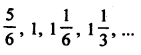Here,

First term = a = 5/6

Common difference = d = 1 – 5/6 = 1/6

Now: an = a + (n – 1)d

3 = 5/6 + (n – 1)1/6

Let nth term is 3

Now, an = a + (n-1)d

3 = 5/6 + (n-1)1/6

n -1 = 13

n = 13 + 1 = 14

Therefore, 3 is the 14th term.

Question 13:

Which term of the AP 21, 18, 15,… is -81?

Solution:

AP is 21, 18, 15, ……

Let nth term -81

Here, a = 21, d = 18 – 21 = -3

an = a + (n – 1)d

-81 = 21 + (n – 1)(-3)

-81 – 21 = (n – 1)(-3)

-102 = (n – 1)(-3)

n = 34 + 1 = 35

Therefore, -81 is the 35th term

Question 14:

Which term of the AP 3, 8, 13, 18, …will be 55 more than its 20th term?

Solution:

Given AP is 3, 8, 13, 18,…

First term = a = 3

Common difference = d = 8 – 3 = 5

And n = 20 and a20 be the 20th term, then

a20 = a + (n – 1)d

= 3 + (20 – 1) 5

= 3 + 95

= 98

The required term = 98 + 55 = 153

Now, 153 be the nth term, then

an = a + (n – 1)d

153 = 3 + (n – 1) x 5

153 – 3 = 5(n – 1)

150 = 5(n – 1)

n – 1 = 30

n = 31

Required term will be 31st term.

Question 15:

Which term of the AP 5, 15, 25,… will be 130 more than its 31st term?

Solution:

AP is 5, 15, 25,…

First term = a = 5

Common difference = d = 15 – 5 = 10

Find 31st term:

a31 = a + (n – 1)d

= 5 + (31 – 1) 10

= 5 + 30 x 10

= 305

Required term = 305 + 130 = 435

Now, say 435 be the nth term, then

an = a + (n – 1)d

435 = 5 + (n – 1)10

435 – 5 = (n – 1)10

n – 1 = 43

n = 44

The required term will be 44th term.

Question 16:

If the 10th term of an AP is 52 and 17th term is 20 more than its 13 th term, find the AP

Solution:

Let a be the first term and d be the common difference, then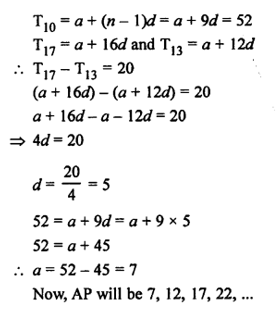Question 17:

Find the middle term of the AP 6, 13, 20,…, 216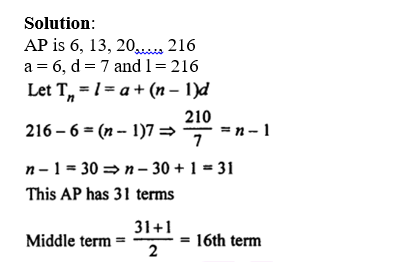a16 = 6 + (16 – 1)7 = 6 + 105 = 111

Therefore, midterm of the AP id 111.

Question 18:

Find the middle term of the AP 10, 7, 4, ………. (-62)

Solution:

AP is 10, 7, 4, …..…, (-62)

a = 10,

d = 7 – 10 = -3, and

l = -62

Now, an = l = a + (n – 1)d

-62 = 10 + (n – 1) x (-3)

-62 – 10 = -3(n- 1)

-72 = -3(n – 1)

Or n = 24 + 1 = 25

Middle term = (25 + 1) /2 th = 13th term

Find the 13th term using formula, we get

a13 = 10 + (13 – 1)(-3) = 10 – 36 = -26

Question 19:

Find the sum of two middle most terms of the AP -4 /3 , -1, -2 /3 , …, $4\frac{1}{3}$ .

Solution:

Given AP is -4 /3 , -1, -2 /3 , …, 13 /3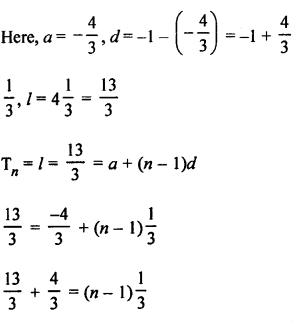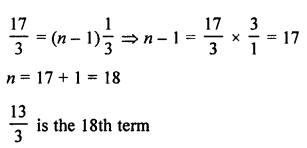Middle terms will be: (18/2)th + (18/2 + 1)th

= 9th + 10th term

Now,

a9 + a10 = a + 8d + a + 9d

= 2a + 17d

= 2(-4/3) + 17(1/3)

= 3

Question 20:

Find the 8th term from the end of the AP 7, 10, 13, ……, 184.

Solution:

Given: AP is 7, 10, 13,…, 184

a = 7, d = 10 – 7 = 3 and l = 184

nth term from the end = l – (n – 1)d

Now,

8th term from the end be

184 – (8 – 1)3 = 184 – 21 = 163

Question 21:

Find the 6th term from the end of the AP 17, 14, 11, …, (-40).

Solution:

Given: AP is 17, 14, 11, …,(-40)

a = 17, d = 14 – 17 = -3, l = -40

6th term from the end = l – (n – 1)d

= -40 – (6 – 1) (-3)

= -40 – (5 x (-3))

= -40 + 15

= -25

Question 22:

Is 184 a term of the AP 3, 7, 11, 15, …..?

Solution:

Given AP is 3, 7, 11, 15, …

a = 3, d = 7 – 3 = 4

Let 184 be the nth term of the AP

an = a + (n – 1)d

184 = 3 + (n – 1) x 4

184 – 3 = (n – 1) x 4

181 /4 = n – 1

n = 181 /4 + 1 = 185/4 (Which is in fraction)

Therefore, 184 is not a term of the given AP.

Question 23:

Is -150 a term of the AP 11, 8, 5, 2,…?

Solution:

Given AP is AP 11, 8, 5, 2,…

Here a = 11, d = 8-11= -3

Let -150 be the nth term of the AP

an = a + (n – 1)d

-150 = 11 +(n-1)(-3)

or n = 164/3

Which is a fraction.

Therefore, -150 is not a term of the given AP.

Question 24:

Which term of the AP 121, 117, 113,… is its first negative term?

Solution:

Let nth of the AP 121, 117, 113,… is negative. Let Tn be the nth term then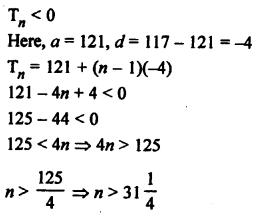Therefore, 32nd term will be the 1st negative term.

Question 25:

Which term of the AP 20, 19 1 /4 , 18 1 /2 , 17 3 /4 , …………. is its first negative term?

Solution: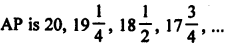a = 20, d = -3/4

Let nth term be the 1st negative term of the AP

an < 0

an = a + (n – 1)d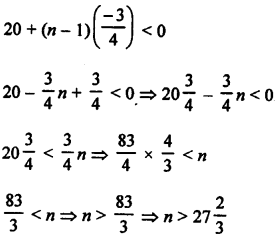Therefore, 28th term will be the 1st negative term.

Question 26:

The 7th term of an AP is -4 and its 13th term is -16. Find the AP.

Solution:

Let us say a be the first term and d be the common difference of an AP

an = a + (n – 1)d

a7 = a + (7 – 1)d

= a + 6d = -4 …………(1)

And a13 = a + 12d = -16 …..…..(2)

Subtracting equation (1) from (2), we get

6d = -16 – (-4) = -12

From (1), a + 6d = -4

a + (-12) = -4

a = -4 + 12 = 8

a = 8, d = -2

AP will be 8, 6, 4, 2, 0, ……

Question 27:

The 4th term of an AP is zero, Prove that its 25th term is triple its 11th term.

Solution:

Let a be the first term and d be the common difference of an AP.

a4 = a + (n- 1)d

= a + (4 – 1)d

= a + 3d

Since 4th term of an AP is zero.

a + 3d = 0

or a = -3d ….(1)

Similarly,

a25 = a + 24d = -3d + 24d = 21d …(2)

a11 = a + 10d = -3d + 10d = 7d ….(3)

From (2) and (3), we have

a25 = 3 x a11

Hence proved.

Question 28:

If the sixth term of an AP is zero then show that its 33rd term is three times its 15th term.

Solution:

Sixth term of an AP is zero

that is a6 = 0

a + 5d = 0

a = -5 d

Now, a15 = a + (n – 1 )d

a + (15 – 1)d = -5d + 14d = 9d

and a33 = a + (n – 1 )d = a + (33 – 1)d = -5d + 32d = 27d

Now, a33 : a12

27d : 9d

3 : 1

Which shows that a33 = 3(a15)

Hence proved.

Question 29:

The 4th term of an AP is 11. The sum of the 5th and 7th terms of this AP is 34. Find its common difference.

Solution:

Let a be the first term and d be the common difference of an AP.

an = a + (n – 1)d

a4 = a + (4 – 1)d = a + 3d

a + 3d = 11 ………(1)

Now, a5 = a + 4d and a7 = a + 6d

Now, a5 + a7 = a + 4d + a + 6d = 2a + 10d

2a + 10d = 34

a + 5d = 17 ……..(2)

Subtracting (1) from (2), we get

2d = 17 – 11 = 6

d = 3

The common difference = 3

Question 30:

The 9th term of an AP is -32 and the sum of its 11th and 13th terms is -94. Find the common difference of the AP.

Solution:

Let a be the first term and d be the common difference of an AP.

nth term = an = a + (n – 1)d

Given: 9th term of an AP is -32 and the sum of its 11th and 13th terms is -94

Now,

a9 = a + 8d = -32 …(1)

a11 = a + 10d

a13 = a + 12d

Sum of 11th and 13th terms:

a11 + a13 = a + 10d + a + 12d

-94 = 2a + 22d

or a + 11d =-47 …(2)

Subtracting (1) from (2) , we have

3d = -47 + 32 = -15

or d = -5

Common difference is -5.

## R S Aggarwal Solutions for Class 10 Maths Chapter 5 Arithmetic Progression Exercise 5A

Class 10 Maths Chapter 5 Arithmetic Progression Exercise 5A is based on following topics:

• Arithmetic Progression introduction
• Arithmetic series
• Finding the general term of an AP
• Finding the nth term from the end of an AP